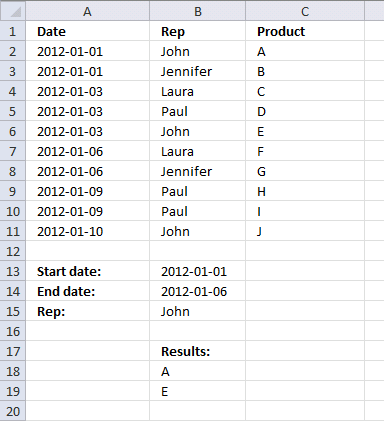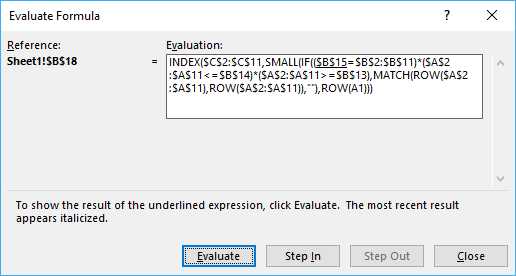Author: Oscar Cronquist Article last updated on August 25, 2017

I have a set of data, like the one you used in the original example that also has a column for the date of the transaction. I would like my Index-type formula to search for both the main item (the rep's name) and also if the date of the transaction falls in the date range).

Start Date: 11/26/2012
End Date: 11/30/2012 (both entered by the user)
Rep: John

Then the results, in each row/column of the 'result' section (INDEX formula results) would show results for John that occurred from 11/26 to 11/30 (including both dates).

Thanks for any help with the formula for that.

JasonArray formula in cell B18:

=INDEX(\$C\$2:\$C\$11, SMALL(IF((\$B\$15=\$B\$2:\$B\$11)*(\$A\$2:\$A\$11<=\$B\$14)*(\$A\$2:\$A\$11>=\$B\$13), MATCH(ROW(\$A\$2:\$A\$11), ROW(\$A\$2:\$A\$11)), ""), ROW(A1)))

Lookup multiple values in different columns and return a single value

S.Babu asks: Dear Oscar, I m working on the below table. ORDER MODEL MATERIAL QTY STATUS BOM a s6 1 […]

### How to create an array formula

1. Copy above array formula
2. Double click on cell E2
3. Paste array formula
4. Press and hold Ctrl + Shift simultaneously
5. Press Enter once
6. Release all keys

How to enter an array formula

Array formulas allows you to do advanced calculations not possible with regular formulas.

### How to copy array formula

1. Select cell E2
2. Copy cell (Ctrl + c)
3. Select cell E3:E20
4. Paste (Ctrl + v)

### Explaining array formula in cell B18

You can easily follow along, select cell B18. Go to tab "Formulas" and click "Evaluate formula" button.Click "Evaluate" button, shown in above picture, to move to next step.

#### Step 1 - Compare value in cell B15 to cell range \$B\$2:\$B\$11

\$B\$15=\$B\$2:\$B\$11

becomes

"John"={"John";"Jennifer";"Laura";"Paul";"John";"Laura";"Jennifer";"Paul";"Paul";"John"}

and returns

{TRUE;FALSE;FALSE;FALSE;TRUE;FALSE;FALSE;FALSE;FALSE;TRUE}

#### Step 2 - Compare start and end date to date column

(\$A\$2:\$A\$11<=\$B\$14)*(\$A\$2:\$A\$11>=\$B\$13)

becomes

({TRUE; TRUE; TRUE; TRUE; TRUE; TRUE; TRUE; FALSE; FALSE; FALSE})*({TRUE; TRUE; TRUE; TRUE; TRUE; TRUE; TRUE; TRUE; TRUE; TRUE})

and returns

{1;1;1;1;1;1;1;0;0;0}

#### Step 3 - If a record is a match return it´s row number

IF((\$B\$15=\$B\$2:\$B\$11)*(\$A\$2:\$A\$11<=\$B\$14)*(\$A\$2:\$A\$11>=\$B\$13),MATCH(ROW(\$A\$2:\$A\$11),ROW(\$A\$2:\$A\$11))

becomes

IF({TRUE; FALSE; FALSE; FALSE; TRUE; FALSE; FALSE; FALSE; FALSE; TRUE}*{1;1;1;1;1;1;1;0;0;0},MATCH(ROW(\$A\$2:\$A\$11),ROW(\$A\$2:\$A\$11))

becomes

IF({1;0;0;0;1;0;0;0;0;0},{1; 2; 3; 4; 5; 6; 7; 8; 9; 10})

and returns

{1;FALSE;FALSE;FALSE;5;FALSE;FALSE;FALSE;FALSE;FALSE}

How to use the IF function

Checks if a logical expression is met. Returns a specific value if TRUE and another specific value if FALSE.

#### Step 4 - Return the k-th smallest value

SMALL(IF((\$B\$15=\$B\$2:\$B\$11)*(\$A\$2:\$A\$11<=\$B\$14)*(\$A\$2:\$A\$11>=\$B\$13),MATCH(ROW(\$A\$2:\$A\$11),ROW(\$A\$2:\$A\$11)),""),ROW(A1))

becomes

SMALL({1; FALSE; FALSE; FALSE; 5; FALSE; FALSE; FALSE; FALSE; FALSE},ROW(A1))

becomes

SMALL({1; FALSE; FALSE; FALSE; 5; FALSE; FALSE; FALSE; FALSE; FALSE},1)

and returns 1.

How to use the SMALL function

The SMALL function lets you extract a number in a cell range based on how small it is compared to the other numbers in the group.

#### Step 5 - Return the value of a cell at the intersection of a particular row and column

INDEX(\$C\$2:\$C\$11,SMALL(IF((\$B\$15=\$B\$2:\$B\$11)*(\$A\$2:\$A\$11<=\$B\$14)*(\$A\$2:\$A\$11>=\$B\$13),MATCH(ROW(\$A\$2:\$A\$11),ROW(\$A\$2:\$A\$11)),""),ROW(A1)))

becomes

INDEX(\$C\$2:\$C\$11,1)

becomes

INDEX({"A"; "B"; "C"; "D"; "E"; "F"; "G"; "H"; "I"; "J"},1)

and returns A in cell B18.

How to use the INDEX function

Gets a value in a specific cell range based on a row and column number.